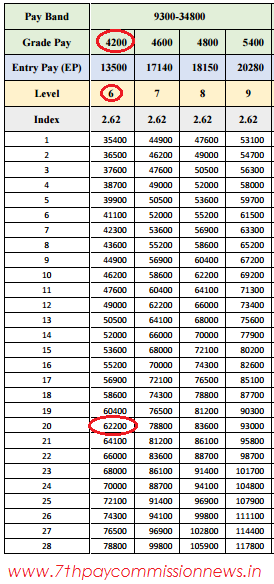## rp

Blogging Tips 2017

### 7th CPC Pay Fixation with examples

Pay Fixation in the New Pay Structure : The fitment of each employee in the new pay matrix is proposed to be done by multiplying his/her basic pay on the date of implementation by a factor of 2.57.
The figure so arrived at is to be located in the new pay matrix, in the level that corresponds to the employee’s grade pay on the date of implementation, except in cases where the Commission has recommended a change in the existing grade pay. If the identical figure is not available in the given level, the next higher figure closest to it would be the new pay of the concerned employee. A couple of examples are detailed below to make the process amply clear.

The pay in the new pay matrix is to be fixed in the following manner:
Step 1: Identify Basic Pay (Pay in the pay band plus Grade Pay) drawn by an employee as on the date of implementation. This figure is ‘A’.
Step 2: Multiply ‘A’ with 2.57, round-off to the nearest rupee, and obtain result ‘B’.
Step 3: The figure so arrived at, i.e., ‘B’ or the next higher figure closest to it in the Level assigned to his/her grade pay, will be the new pay in the new pay matrix. In case the value of ‘B’ is less than the starting pay of the Level, then the pay will be equal to the starting pay of that level
Example I
i. For example an employee H is presently drawing Basic Pay of Rs. 55,040 (Pay in the Pay Band Rs. 46340 + Grade Pay Rs. 8700 = Rs. 55040). After multiplying Rs. 55,040 with 2.57, a figure of Rs. 1,41,452.80 is arrived at. This is rounded off to Rs. 1,41,453.
ii. The level corresponding to GP 8700 is level 13, as may be seen from Table 4, which gives the full correspondence between existing Grade Pay and the new Levels being proposed.
iii. In the column for level 13, the figure closest to Rs.1,41,453 is Rs.1,41,600.
iv. Hence the pay of employee H will be fixed at Rs.1,41,600 in level 13 in the new pay matrix as shown below:As part of its recommendations if Commission has recommended any up gradation or downgrade in the level of a particular post, the person would be placed in the level corresponding to the newly recommended grade pay.
Example II
i. Take the case of an employee T in GP 4200, drawing pay of Rs. 20,000 in PB-2. The Basic Pay is Rs. 24,200 (20,000+4200). If there was to be no change in T’s level the pay fixation would have been as explained in Example I above. After multiplying by 2.57, the amount fetched viz., Rs. 62,194 would have been located in Level 6 and T’s pay would have been fixed in Level 6 at Rs. 62,200.ii. However, assuming that the Commission has recommended that the post occupied by T should be placed one level higher in GP 4600. T’s basic pay would then be Rs 24,600 (20000 + 4600). Multiplying this by 2.57 would fetch Rs. 63,222.
iii. This value would have to be located in the matrix in Level 7 (the upgraded level of T).
iv. In the column for Level 7 Rs. 63,222 lies between 62200 and 64100. Accordingly, the pay of T will be fixed in Level 7 at Rs.64,100.